# How to measure the performance of the language model ?

1. While building language model, we try to estimate the probability of the sentence or a document.
2. Given sequences(sentences or documents) like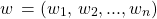3. Language model(bigram language model) will be :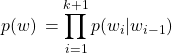for each sequence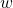given by above equation.

4. Once we apply Maximum Likelihood Estimation(MLE), we should have a value for the term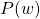.
5. Perplexity is the inverse of square root of likelihood. So lesser the perplexity, better is the model. Note that square root in case of bigram language model,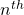root in case of n-gram model. For more explanation on perplexity, visit this question